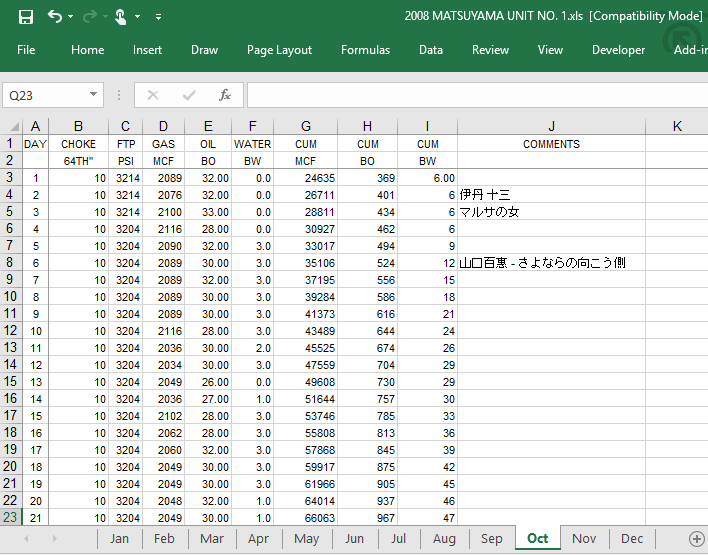# Processing Excel Data with Powershell

When a new customer signs up for Productioneer, Mi4 imports their historical data from their previous system into their new Productioneer environment so that they can have a comprehensive view of their assets. Typically our customers are switching from another system like GRT, FieldDirect, Merrick, etc and the data is relatively straightforward for us to import.

Sometimes we have clients that weren’t using a field data capture system at all and were keeping track of their production in Excel. Normally these companies only have 20 or so wells and manually manipulating the Excel spreadsheets into an importable format isn’t too labor intensive.

An exception to this rule was a new client we on-boarded, let’s call them Hakamada Resources because their actual name isn’t really pertinent to the blog post and this Juzo Itami movie I just watched was really good.

At the time of their switch to Productioneer Hakamada Resources had over 100 wells and historical data stretching back over 10 years – all in Excel. That wouldn’t be so bad but the kicker was that the data was stored monthly. Each excel file was one full year and each sheet was a month.Another team member, let’s call him David because that’s his name, and I were working on the Hakamada Resources implementation. When we saw the data format we threw out a couple of ideas.

Idea #1: Import each excel worksheet separately – ~100 wells x 10 years x 12 months = let’s tell Mr. Hakamada we couldn’t do it and go eat some どら焼き

Idea #2: Cut and paste each excel sheet on to a single master sheet and import the master sheet = tell Mr. Hakamada we’d be done in a couple of months and we would need to charge him extra because we wore out the Ctrl, c, v, shift, and arrow keys on 252 keyboards from 秋葉原.

Idea #3: Write a VBA script to automate idea #2 – a viable solution, in fact it might be the best one. The reason we did not go with it is because we did not know how to address and reference of all the files and sheets in VBA off the top of our heads. We did know how in PowerShell.

Idea #4: Write a PowerShell script to automate idea #2 –After some thought David and I decided option #4 was best. We called it a day, ate some どら焼きand started on the script the next day.

As I said earlier, each sheet was a month and luckily they followed the same format most of the time. The first 4 characters of the filename were the year followed by the well name. The sheet name was the 3 letter abbreviation of the month (Feb, Aug, Jul, etc) and the data ranged from cells A3 to I33.
We put all of the files in one folder, and wrote a script to open each sheet in each file and paste it into a new sheet along with the meta data gathered from the filename and worksheet name:

$Files = Get-ChildItem 'C:\your folder\where\all\of those\Excel\files are' | ?{$_.Extension -Match "xls?"} | Select -ExpandProperty FullName
$Excel = New-Object -ComObject Excel.Application$Excel.Visible = $True$Excel.DisplayAlerts = $False$Dest = $Excel.Workbooks.Add()$startcol = 1
ForEach($File in$Files){
$Source =$Excel.Workbooks.Open($File,$true,$true)$baseName = [System.IO.Path]::GetFileNameWithoutExtension($File)$split = $baseName.Split("{ }")$month = 0
foreach($worksheet in$Source.WorkSheets)
{
if($worksheet.Name -like "Jan"){$month = 1} if($worksheet.Name -like "Feb"){$month = 2} if($worksheet.Name -like "Mar"){$month = 3}
if($worksheet.Name -like "Apr"){$month = 4} if($worksheet.Name -like "May"){$month = 5} if($worksheet.Name -like "Jun"){$month = 6}
if($worksheet.Name -like "Jul"){$month = 7} if($worksheet.Name -like "Aug"){$month = 8} if($worksheet.Name -like "Sep"){$month = 9}
if($worksheet.Name -like "Oct"){$month = 10} if($worksheet.Name -like "Nov"){$month =11} if($worksheet.Name -like "Dec"){$month = 12}
if($month -ne 0 ) {$SheetName = $worksheet.Name [void]$worksheet.Range("A3","K33").Copy()
[void]$Dest.Activate() If(($Dest.ActiveSheet.UsedRange.Count -eq 1) -and ([String]::IsNullOrEmpty($Dest.ActiveSheet.Range("A3").Value2))){ [void]$Dest.ActiveSheet.Range("A3").Select()
}Else{
[void]$Dest.ActiveSheet.Range("A$(($Dest.ActiveSheet.UsedRange.Rows|Select -last 1).row+1)").Select() } [void]$Dest.ActiveSheet.Paste()
$USedrange =$Dest.ActiveSheet.UsedRange.Rows.Count
$Dest.ActiveSheet.Range("M" +$startcol,"M" + $USedrange).Value2 =$baseName
$Dest.ActiveSheet.Range("N" +$startcol,"N" + $USedrange).Value2 =$SheetName
for($i =$startcol;$i -le$USedrange;$i++) {$Dest.ActiveSheet.Range("O"+ $i).Value2 = [string]$month+ "/" +[string]$Dest.ActiveSheet.Range("A" +$i).Value2 + "/" +[string]$split }$startcol = $USedrange + 1 }$month = 0
}
$Source.Close()$Dest.SaveAs("C:\the folder\you want to\save the combined\Excel file\to\your file name.xlsx",51) #put this here so that you save your progress in case something interrupts the loop
#this way you can pickup where you left off
}
$Dest.SaveAs("C:\the folder\you want to\save the combined\Excel file\to\your file name.xlsx",51)$Dest.close()
\$Excel.Quit()

The PowerShell script took a while to cycle through all of the files. It also opens and runs an excel instance on your workstation. You can still use your workstation while the script is executing but copying something to your clipboard (even from a different application) will most likely cause what you copied to be pasted into the target excel sheet. Because of these factors, I recommend executing the script on a VM or a dedicated workstation that you do not need to use for half an hour.

After all of Hakamada Resources’ data was in one nice clean (and large) excel sheet, we could import it into Productioneer. The rest of the Hakamada on-boarding was pretty straightforward and they are happy Productioneer customer today.

While the Hakamada data was not the easiest historical data to import into Productioneer it was far from the worst thanks to the PowerShell solution and the uniformity and consistency of the Hakamada spreadsheets.